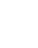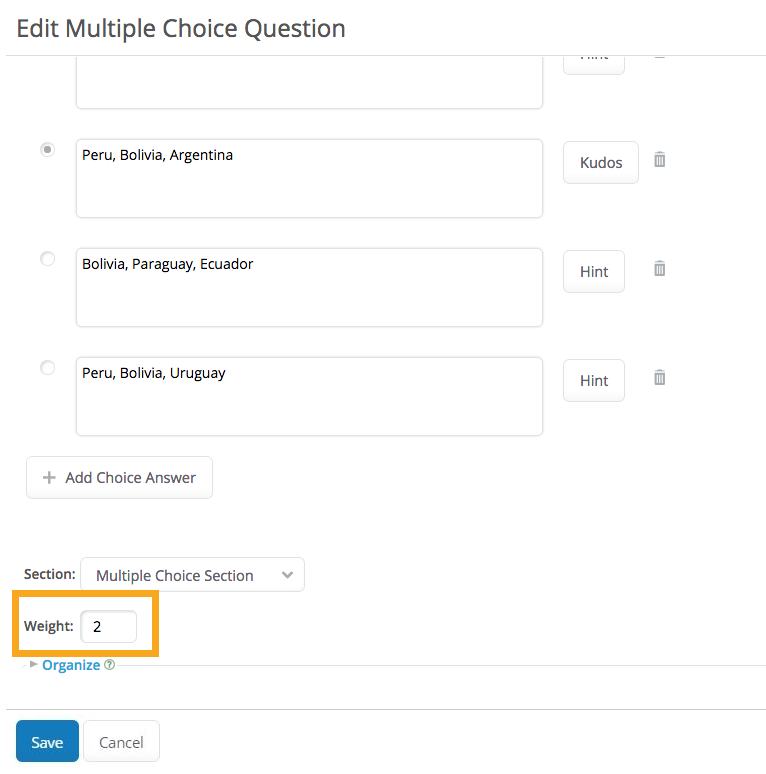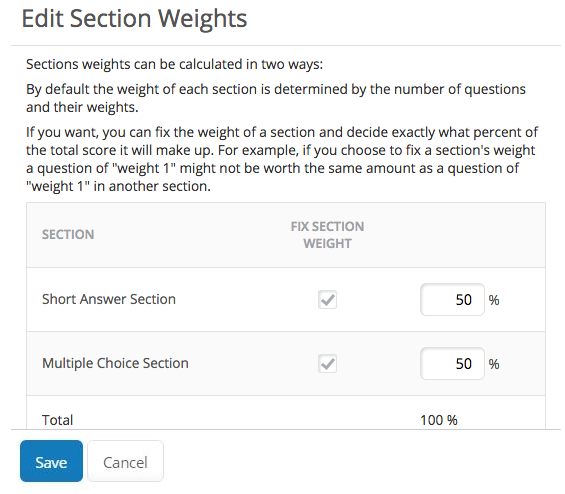# What are my options for weighting my Assessment?

## Question

How do I use weighting in my Assessments?

In your Assessments you can assign Section and/or Question weights, which dictate questions' value in the Assessment as a whole.

Question weights

You can assign an individual question a weight, which will affect how much value the Question has within its Section (if you're using Sections in your Assessment!), or within the entire Assessment.

By default, each question is given a weight of 1. In the editing area of a question, you'll see a field for the question's weight.Or, in the Assessment's editing overview area, you can click the question's Weight button to adjust its weight.Weight adjustments must be positive and must be in whole number increments (e.g. it's not possible to give a Question a weight of .5).

In the example pictured above, the Multiple Choice Section has 2 questions: the first question is worth 2 points, and the second is worth 1 point. This means that the first question will be worth twice what the second question is worth, or ~66% of the entire section.

Section weights

If you have multiple Sections in your Assessment, you can weight each Section, to make that Section's questions worth more or less in the grand scheme of the Assessment.

To weight your Sections, click the Weight button next to one of the Sections. This will open a dialog box where you can distribute weights to each Section.

By default the weight of each Section is determined by the number of questions in the Section and their weights.

If you'd like to manually adjust the weights, just click the "Fix Section Weight" checkbox for the Section, and you'll be able to enter in new percentage values for the Section's weight.In the example pictured above, each section is worth 50% of the Assessment's entire value.

See an example

To see how this all plays out, let's use the example pictured in this article and calculate how the Assessment will be scored.

• Section 1: Short Answer has a weight of 50%, and it contains one question, worth 1 point.
• Section 2: Multiple Choice has a weight of 50%, and it contains two questions, one worth 2 points, and one worth 1 point.

If the student gets all of the questions right:

Section 1: 1/1 (points earned out of points available) x 50% (Section's weight) = 50%

Section 2: 3/3 x 50% = 50%

Complete Assessment: 50% (Section 1) + 50% (Section 2) = 100%

If the student misses some:

Section 1: .5/1 x 50% = 25%

Section 2: 2/3 x 50% = 33.33%

Complete Assessment: 25% + 33% = 58.33%

Contributors